# Bernoulli's Principle

A key concept in fluid dynamics, Bernoulli’s principle relates the pressure of a fluid to its speed. When the speed of a fluid increases, the pressure decreases, and vice versa. Bernoulli's equation can be used to approximate these parameters in water, air or any fluid that has low viscosity. The principle is named after Swiss mathematician and physicist Daniel Bernoulli who first published it in his book Hydrodynamica in 1738.

Derived from the principle of energy conservation, Bernoulli's equation tells us that the sum of the pressure, kinetic and potential energy along a streamline in a fluid flow must remain constant. The equation applies to the case of flow that is steady (not changing), has constant density and is inviscid (where the viscosity of the fluid is close to zero). The Bernoulli equation states that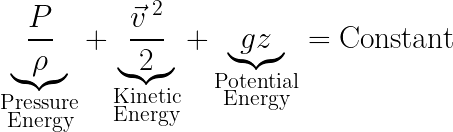where P is pressure, ρ is density, v is velocity, z is elevation/height above a reference plane, and g is gravitational acceleration. Understanding how Bernoulli's principle works enables engineers to leverage the nature of fluid pressure so their designs function correctly, efficiently and safely.

Engineers make a world of difference!

Engineers use Bernoulli's principle in a wide range of applications in engineering fluid dynamics, from aerospace wing design, designing pipes for hydroelectric plants to designing medical equipment.Bernoulli's Principle CurriculaTake a plunge into the resources featured here, by grade band, to help your students make sense of the fluid dynamics phenomenon of Bernoulli's Principle!

• Coming Soon!

• Coming Soon!

• Fun with Bernoulli
Fun with Bernoulli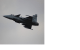While we know air exists around us all the time, we usually do not notice the air pressure. During this activity, students use Bernoulli's principle to manipulate air pressure so its influence can be seen on the objects around us.

• Windy Tunnel
Windy TunnelThrough this activity, Bernoulli's principle as it relates to winged flight is demonstrated. Student pairs use computers and an online virtual wind tunnel to see the influence of camber and airfoil angle of attack on lift. Activity and math worksheets are provided.

• May the Force Be with You: Thrust
May the Force Be with You: Thrust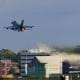Students study how propellers and jet turbines generate thrust. This lesson focuses on Isaac Newton's third law of motion for every action there is an equal and opposite reaction.

• Can You Take the Pressure?
Can You Take the Pressure?Students are introduced to the concept of air pressure. They explore how air pressure creates force on an object. They study the relationship between air pressure and the velocity of moving air.

• Building Tetrahedral Kites
Building Tetrahedral KitesWorking in teams of four, students build tetrahedral kites following specific instructions and using specific materials. They use the basic processes of manufacturing systems – cutting, shaping, forming, conditioning, assembling, joining, finishing, and quality control – to manufacture complete tetr...

• Bernoulli's Principle
Bernoulli's Principle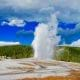Students learn about the relationships between the components of the Bernoulli equation through real-life engineering examples and practice problems.

• Archimedes' Principle, Pascal's Law and Bernoulli's Principle
Archimedes' Principle, Pascal's Law and Bernoulli's Principle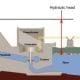Students are introduced to Pascal's law, Archimedes' principle and Bernoulli's principle. Fundamental definitions, equations, practice problems and engineering applications are supplied.

• Tippy Tap Plus Piping
Tippy Tap Plus Piping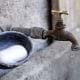In this activity, student teams design, prototype and test piping systems to transport water from a storage tank to an existing Tippy Tap hand-washing station, thereby creating a more efficient hand-washing station. Through this example service-learning engineering project, students learn basic flui...

• A Shot Under Pressure
A Shot Under PressureStudents use their understanding of projectile physics and fluid dynamics to find the water pressure in water guns. By measuring the range of the water jets, they are able to calculate the theoretical pressure.

More Popular Topics to explore
Free K-12 standards-aligned STEM curriculum for educators everywhere.
Find more at TeachEngineering.org